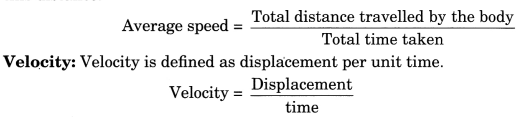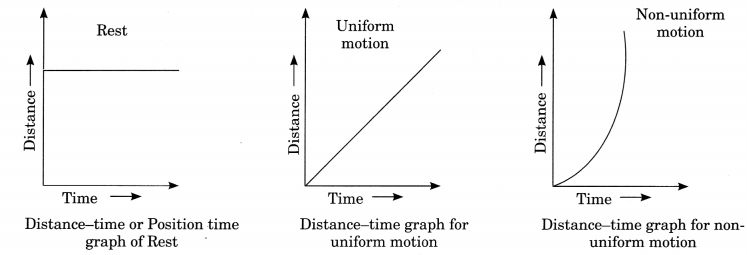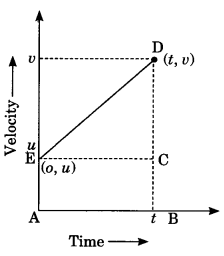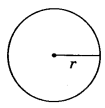On this page, you will find Motion Class 9 Notes Science Chapter 8 Pdf free download. CBSE NCERT Class 9 Science Notes Chapter 8 Motion will seemingly help them to revise the important concepts in less time.

## CBSE Class 9 Science Chapter 8 Notes Motion

### Motion Class 9 Notes Understanding the Lesson

1. Rest: A body is said to be at rest if its position does not change with respect to a fixed point taken as a reference point in its surrounding with the passage of time.

2. Motion: A body is said to be in motion if its position is changes with respect to a fixed point taken as a reference point in its surrounding with the passage of time.

3. Scalar quantity: A physical quantity which is described completely by its magnitude only, is called a scalar quantity.

4. Vector quantity: A physical quantity that has magnitude as well as direction is called a vector quantity. Distance: The total path length travelled by a body in a given interval of time is called distance. Displacement: The shortest distance measured from initial to the final position of an object is known as displacement.

5. Difference between distance and displacement

 Distance Displacement 1.   It is the actual length of the path covered by a moving body. 2.   It is always positive or zero. 3.   It is a scalar quantity. 1.    It is the shortest distance measured between the initial and final position. 2.    It may be positive, negative or zero. 3.    It is a vector quantity.

SI unit of distance and displacement is metre (m).

6. Uniform motion: A body moving in straight line has a uniform motion if it travels equal distance in equal intervals of time.

7. Non-uniform motion: A body has a non-uniform motion if it travels unequal distances in equal intervals of time.

8. Speed: The speed of a body is defined as distance travelled by it per unit time.Speed is scalar quantity and SI unit of speed is m/s.

9. Average speed: It is defined as the total distance travelled by a body divided by the total time taken to cover this distance.SI unit of speed and velocity is same.

Difference between speed and velocity

 Speed Velocity 1.   Speed is the ratio of distance and time. 2.   Speed is always positive. 3.   Speed is a scalar quantity. 1.  Velocity is ratio of displacement and time. 2.  Velocity may be negative or positive. 3.  Velocity is a vector quantity.

10. Acceleration: The rate of change of velocity of a body with respect to time is called its acceleration.

$$\text { Acceleration }=\frac{\text { Change in velocity }}{\text { time taken }}$$
$$a=\frac{v-u}{t}$$

Here, u – initial velocity
v = final velocity
t = time

11. Acceleration is a vector quantity and its SI unit is m/s2.

12. If a body is travelling with uniform acceleration then its average velocity can be expressed as

$$v_{\text {avg }}=\frac{u+v}{2}$$
Here, u = initial velocity
v – final velocity

13. If the speed of a body is continuously increasing, the body is said to be continuously accelerating. If the speed of body is continuously decreasing, the body is said to be retarding.

14. Graphical Representation of Motion
Distance-time graph (Position time graph)15. Slope of velocity-time graph gives speed and velocity

16. velocity-time graph17. Slope of velocity-time graph gives distance and displacement.

18. Equation of motion by graphical method
Let us consider a body moving with acceleration a where u is initial velocity and v is final velocity, s is displacement of the object and t is time interval.(i) v = u + at
We know that slope of v – t graph gives acceleration so slope
$$=a=\frac{v-u}{t-o}$$
$$\begin{array}{l} a=\frac{v-u}{t} \\ v=u+\text { at } \end{array}$$(ii) $$s=u t+\frac{1}{2} a t^{2}$$
We know that area under v -1 graph gives displacement.
Area = s = area of triangle CDE + area of rectangle ABCE
$$s=u t+\frac{1}{2} \times t \times(v-u)$$
Putting the value of v – u.
$$s=u t+\frac{1}{2} \mathrm{at}^{2}$$

(iii) v2 – u2 = 2as
a where u is initial velocity and v is final velocity, s is displacement of the object and t is time interval.
$$s=\frac{1}{2} \times(v+u) \times t$$
$$\text { from } 1\left(t=\frac{v-u}{a}\right)$$
Putting the value of t.
v2 – u2 = 2as

19. Uniform circular motion
When an object moves in a circular path with uniform speed, its motion is called uniform circular motion.
$$v=\frac{2 \pi r}{t}$$If a body is moving in a circular path and completes one round (2πr distance) in time, speed is given by
Uniform circular motion is accelerated motion.

### Class 9 Science Chapter 8 Notes Important Terms

State of rest: A body is said to be at rest if it does not change its position with respect to a fixed point taken as a reference point in its surroundings with passage of time.

State of motion: A body is said to be in motion if it changes its position with respect to a fixed point as a reference point in its surroundings with the passage of time.

Scalar quantity: A physical quantity which is described completely by its magnitude only, is called scalar quantity.

Vector quantity: A physical quantity which has magnitude as well as direction and obeys the vector addition is called vector quantity.

Distance: The total path length travelled by a body in a given interval of time is called distance.

Displacement: The shortest distance measured from initial to the final position of an object is known as displacement.

Uniform motion: A body moving in a straight line has a uniform motion, if it travels equal distance in equal intervals of time.

Non-uniform motion: A body has a non-uniform motion, if it travels unequal distances in equal intervals of time.

Speed: The speed of a body is defined as distance travelled by it per unit time.

Velocity: Velocity is defined as displacement per unit time.

Acceleration: The rate of change of velocity of a body with respect to time is called its acceleration.

Retardation: When acceleration of a body is opposite to its velocity, it is called retardation.

Uniform circular motion: When an object is moving in a circular path with a constant speed, the motion of the object is said to be uniform circular motion.